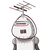# a% of b is equal to b% of a write true or false. and explain how​

a% of b is equal to b% of a write true or false. and explain how​

### 1 thought on “a% of b is equal to b% of a write true or false. and explain how​”

1.true

let take a be 10% and b be 100